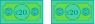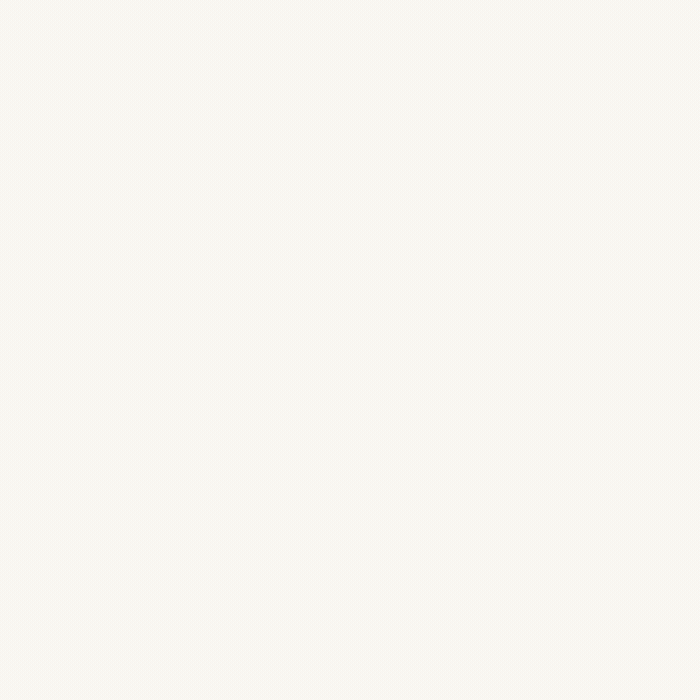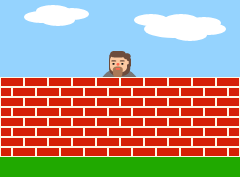A Better Fence

Ms. Price pays you \$20 to mow her yard. After seeing you did such a good job, Mr. Sherman asks if you would mow his yard, too. Mr. Sherman's yard is twice as long and twice as wide as Ms. Price's yard, so he says he'll pay you twice as much.Does Mr. Sherman's math make sense? How much should you charge him?

Let's look at how perimeter and area change as the dimensions of a shape are changed.

All sides have length $x$ in the square below.The perimeter of the square is $x + x + x + x = 4x$.
The area of the square is $x \cdot x = x^{2}$.

Now let's double the length of each side.The perimeter is now $2x + 2x + 2x + 2x = 8x$.

After doubling each side length, the perimeter doubled from $4x$ to $8x$. Length is a one-dimensional measurement, so we multiply by our scale factor of 2 once. The original length of each side was multiplied by $2$, so we multiply the original perimeter by $2$ as well.

The area is now $2x \cdot 2x = 4x^{2}$.After doubling each side length, the area quadrupled from $x^{2}$ to $4x^{2}$. Area is a two-dimensional measurement, so we multiply by our scale factor twice. The original length of each side was multiplied by $2$, so we have to multiply the original area by $2 \cdot 2 = 4$.

Today's Challenge

Pete and Dave are neighbors. They use identical bricks to build fences around their square-shaped yards.Pete uses 1,000 bricks to build his fence.

Dave wants a better fence, so his is three times thicker and three times taller than Pete's. Since his yard is larger, the area enclosed by Dave's fence is also nine times that of Pete's.

How many bricks does Dave use to build his fence?

×